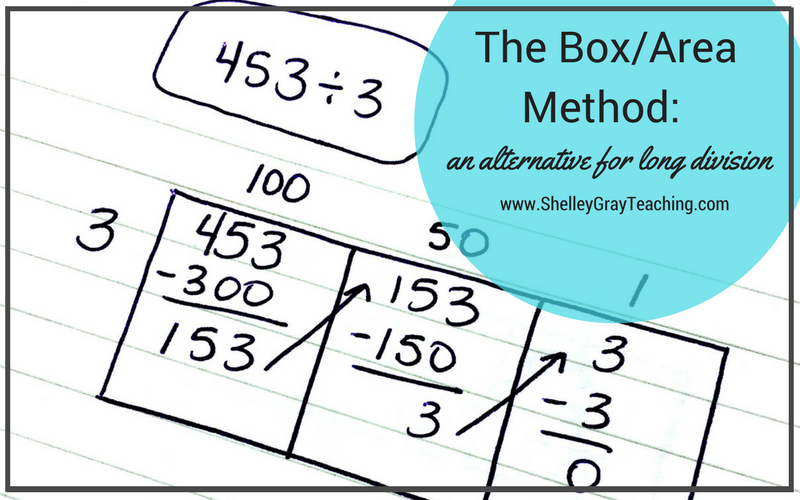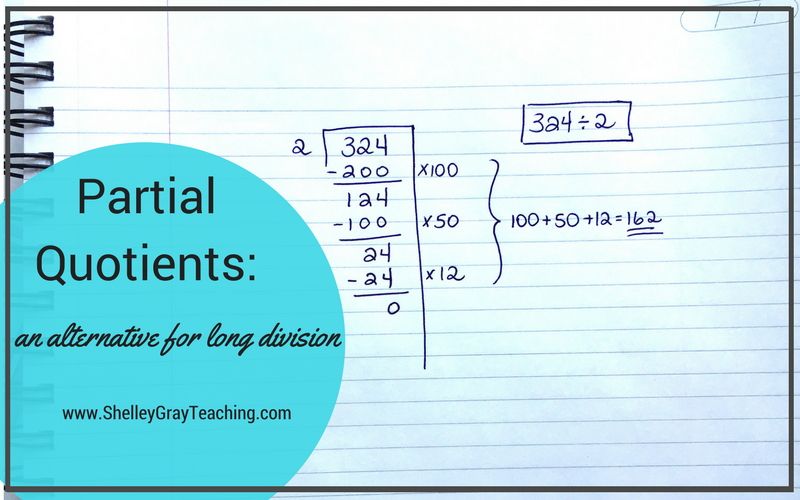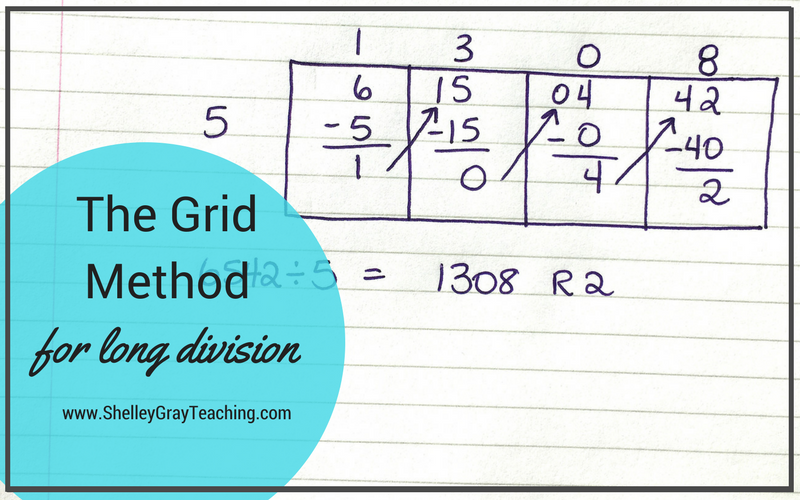# Effective Alternatives for Long Division

Long division is often identified as the most difficult math concept to teach. If we as teachers struggle with teaching long division, then our students will surely struggle with learning it.

One of the main reasons that traditional long division is so hard to learn is that a correct answer depends on a memorized series of steps – divide, multiply, subtract, bring down. If a student forgets which step to do and when to do it, there is a very high chance that he will end up with an incorrect answer.

This is why it is ESSENTIAL to teach strategies, rather than steps.

Strategies encourage REAL UNDERSTANDING. This is such an important part of math. We want our students to really understand what they are doing, and to know that there are different ways to come up with a correct answer.

In this article, I’ll discuss three different alternatives to traditional long division. I encourage you to focus on the first two, as they are very mental math focused.

Ready to get serious about teaching multi-digit division? Check out the Long Division Station HERE.

The Box/Area Method

The Box Method or Area Model is a mental math based approach that will enhance number sense understanding. If you plan on teaching the Partial Quotients strategy (which I will discuss next), this is an excellent way to introduce it.

Students solve the equation by subtracting multiples until they get down to 0, or as close to 0 as possible. For example, in the example below we took out 100 groups of 3, then 50 groups of 3, and then 1 more group of 3 to make a total of 151 groups of 3 taken out of the dividend. Please head over to THIS POST for a very detailed explanation of this strategy, complete with pictures.Partial Quotients

Partial Quotients is a “must-teach” strategy for multi-digit division. If you teach the Box/Area Method first, this is a very natural progression.

When we use the partial quotients strategy, we set up the equation similarly to how a traditional long division equation is set up. The difference here is that we take out multiples of the divisor until we get down to 0, or as close to 0 as we can. Please see THIS POST for a very detailed explanation of this strategy, complete with lots of pictures.The Grid Method

The Grid method is NOT a mental math based approach. This means that if you have students who are struggling with multi-digit division, you should focus on the previous two strategies that I described rather than moving on to this one. The Grid Method can be used for students who are ready for a challenge, or as an introduction if you plan on teaching traditional long division. Some students will find the grid very helpful in organizing their thinking.

When we use the grid method we just organize the digits from the equation into a grid. For a complete, detailed explanation of how to perform the grid method, along with lots of pictures, please see THIS BLOG POST.NEXT STEPS:

••